Tamilnadu State Board New Syllabus Samacheer Kalvi 12th Maths Guide Pdf Chapter 10 Ordinary Differential Equations Ex 10.7 Textbook Questions and Answers, Notes.

Tamilnadu Samacheer Kalvi 12th Maths Solutions Chapter 10 Ordinary Differential Equations Ex 10.7

Question 1.
Solve the following Linear differential equations.
cos x $$\frac { dy }{ dx }$$ + y sin x = 1
Solution:
The given differential equation can be written as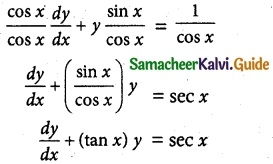This is the form $$\frac { dy }{ dx }$$ + py = Q
where P = tan x
Q = sec x
Thus, the given differential equation is linear.
I.F = e∫ pdx = e∫ tan x dx = elog (sec x) = sec x
So, the required solution is given by
[y × I.F] = ∫ [Q × IF] dx + c
y × sec x = ∫ sec x × sec x dx + c
y sec x = ∫ sec² x dx + c
y sec x = tan x + c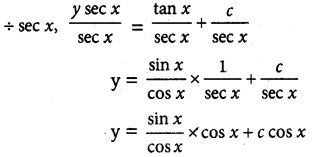= sin x + c cos x
y = sin x + c cos x is the required solution.Question 2.
(1 – x²)$$\frac { dy }{ dx }$$ – xy = 1
Solution:
The given differential equation can be written as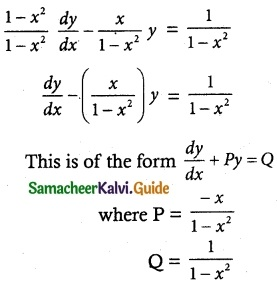Thus, the given differential equation is linear.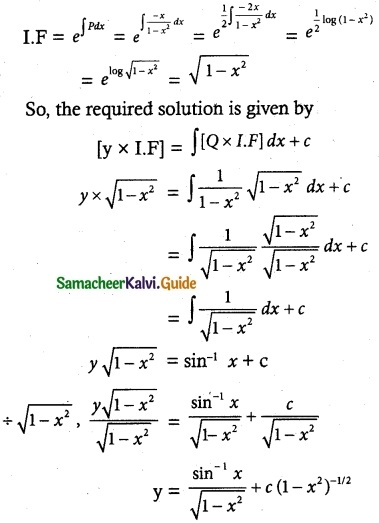Which is a required solution.Question 3.
$$\frac { dy }{ dx }$$ + $$\frac { y }{ x }$$ = sin x
Solution:
The given differential equation can be written as
$$\frac { dy }{ dx }$$ + ($$\frac { 1 }{ x }$$)y = sin x
This is of the form $$\frac { dy }{ dx }$$ + Py = Q
where P = $$\frac { 1 }{ x }$$
Q = sin x
Thus, the given differential equation is linear.
I.F= e∫ pdx = e∫ $$\frac { 1 }{ x }$$ dx = elog x = x
So, the required solution is given by
yx I.F = ∫ (Q × I.F) dx + c
yx = ∫ sin x × x dx + c
= x (-cos x) – (1) (-sin x) + c
yx = -x cos x + sin x + c
yx + x cos x = sin x + c
(y + cos x) x = sin x + c is a required solution.Question 4.
(x² + 1)$$\frac { dy }{ dx }$$ + 2xy = $$\sqrt { x^2+4 }$$
Solution:
The given differential equation may be written as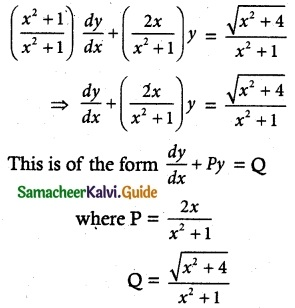Thus, the given differential equation is linear.
I.F= e∫ pdx = e∫ $$\frac { 2x }{ x^+1 }$$ dx = elog (x² + 2) = x² + 1
So, the required solution is given by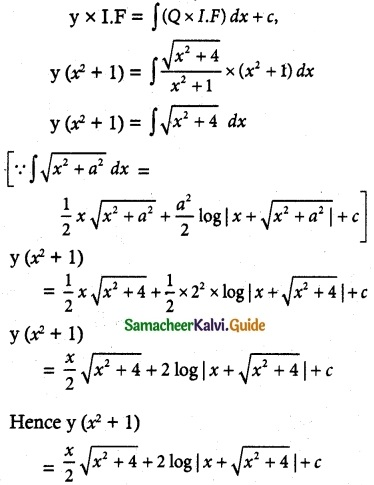Is the required solution.

Question 5.
(2x – 10y³) dy + y dx = 0
Solution:
The given differential equation may be written as
y dx = -(2x – 10y³) dy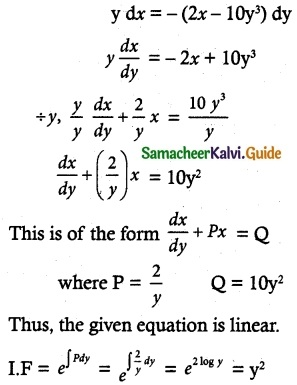So, the required solution is
x × I.F = ∫ (Q × I.F)dy + c
xy² = ∫ 10 y² × y² dy + c
= ∫ 10 y4 dy + c
= $$\frac { 10y^5 }{ 5 }$$ + c = 2y5 + c
xy² = 2y5 + c is a required solution.Question 6.
x sin x $$\frac { dy }{ dx }$$ + (x cos x + sin x) y = sin x
Solution:
The given differential equation can be written as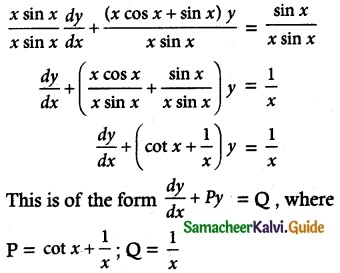Thus, the given differential equation is linear.
I.F = e∫ pdx = e∫ (cot x + 1/x)dx = elog sin x + log x
= elog (x sin x) = x sin x
So, the solution of the given differential equation is given by
y × I.F = ∫(Q × I.F) dx + c
y (x sin x) = ∫ $$\frac { 1 }{ x }$$ x sin x dx + c
= ∫ sin x dx + c
y (x sin x) = -cos x + c
xy sin x + cos x = c is the required solution.Question 7.
Solve (y – esin-1x) $$\frac { dx }{ dy }$$ + $$\sqrt { 1-x^2 }$$ = 0
Solution: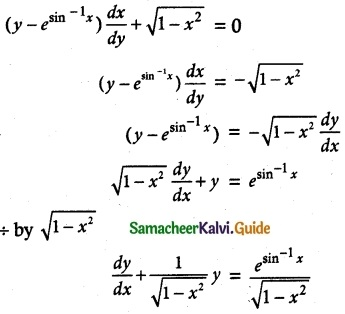Thus, the given differential equation is Linear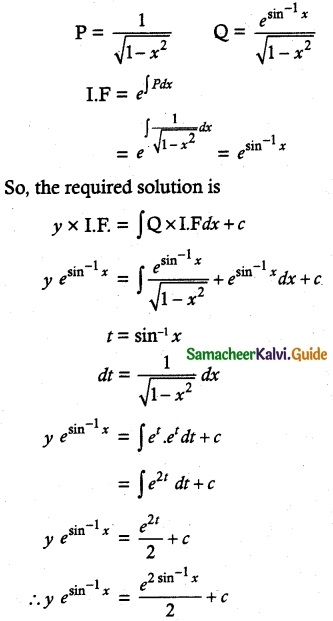Question 8.
$$\frac { dy }{ dx }$$ + $$\frac { y }{ (1-x)√x }$$ = 1 – √x
Solution:
The given linear differential equation is of the form
$$\frac { dy }{ dx }$$ + py = Q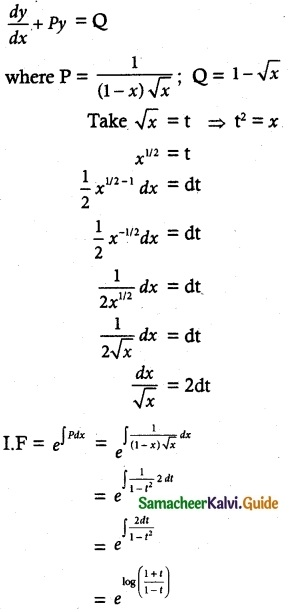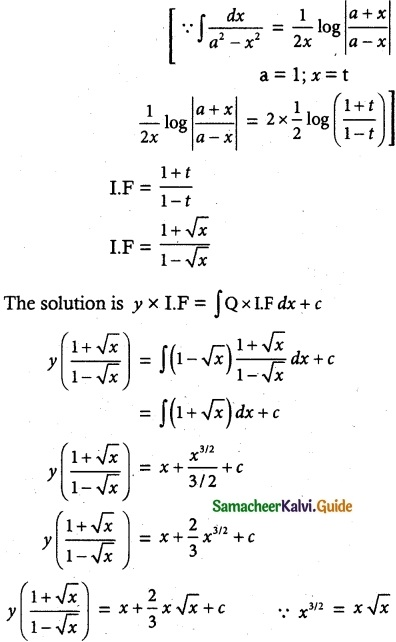Question 9.
(1 + x + xy²) $$\frac { dy }{ dx }$$ + (y + y³) = 0
Solution: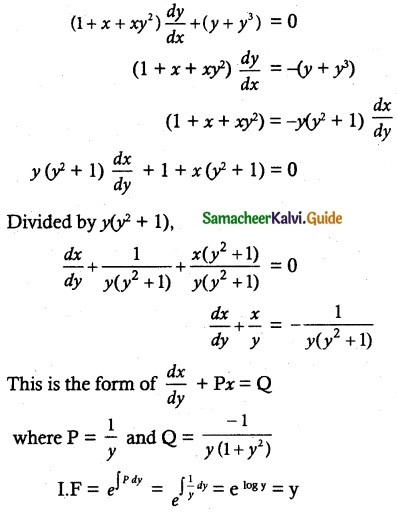So, the solution of the equation is given by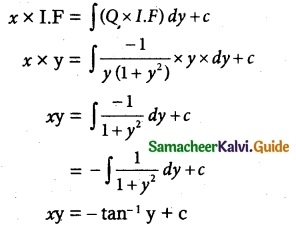xy + tan-1 y = c
Which is the required solution.

Question 10.
$$\frac { dy }{ dx }$$ + $$\frac { y }{ x log x }$$ = $$\frac { sin 2x }{ log x }$$
Solution:
The given differential equation may be written as
$$\frac { dy }{ dx }$$ + $$\frac { 1 }{ x log x }$$y = $$\frac { sin 2x }{ log x }$$
This is of the form $$\frac { dy }{ dx }$$ + Py = Q
Where P = $$\frac { 1 }{ x log x }$$; Q = $$\frac { sin 2x }{ log x }$$
Thus, the differential equation is linear.
I.F = e∫pdx
= e∫ $$\frac { 1 }{ x log x }$$ dx
= e∫ $$\frac { 1 }{ t }$$ dt
= elog t
= log x
So, the solution of the given differential equation is
y × I.F = ∫ (Q × I.F) dx + c
y × log x = ∫ $$\frac { sin 2x }{ log x }$$ × log x dx + c
= ∫ sin 2x dx + c
y log x = $$\frac { -cos 2x }{ 2 }$$ + c
y log x + $$\frac { cos 2x }{ 2 }$$ = c is a required solution.Question 11.
(x + a) $$\frac { dy }{ dx }$$ – 2y = (x + a)4
Solution: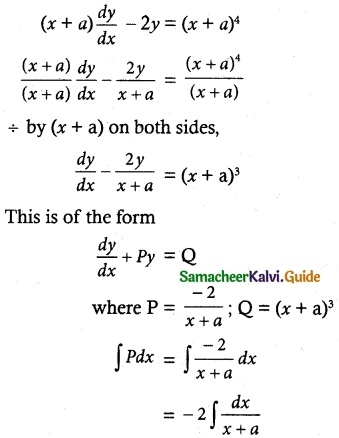= -2 log (x + a)
= log (x + a)-2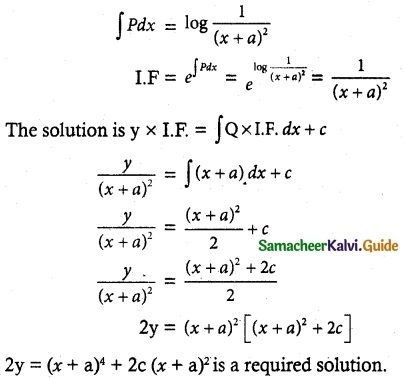Question 12.
$$\frac { dy }{ dx }$$ = $$\frac { sin^x }{ 1+x^3 }$$ – $$\frac { 3x^2 }{ 1+x^3 }$$y
Solution:
The equation can be written as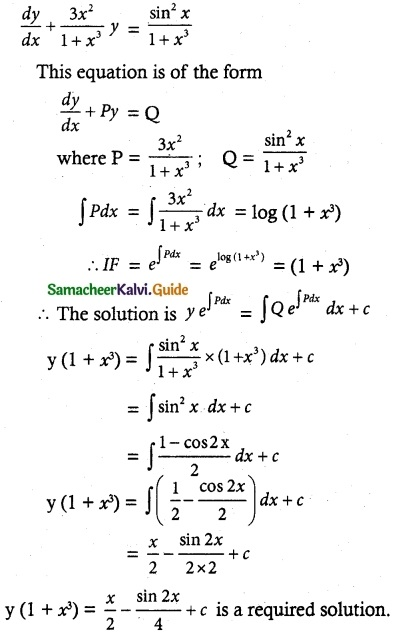Question 13.
x $$\frac { dy }{ dx }$$ + y = x log x
Solution:
The given differential equation may be written as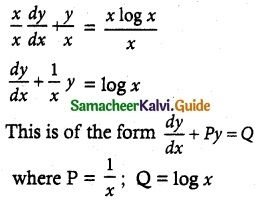Thus, the given differential equation is linear.
I.F = e∫pdx = e∫$$\frac { 1 }{ x }$$ dx = elog x = x
So, the solution of the given differential equation is given by
y × I.F = ∫(Q × I.F)dx + c
yx = ∫ log x x dx + c
yx = ∫ x log x dx + c
u = log x ∫dv = ∫x dx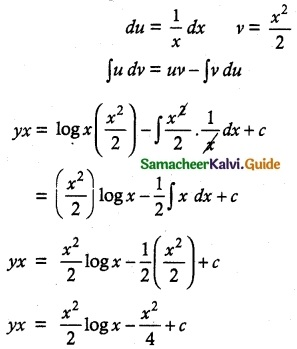Multiply by 4
4yx = 2x² log x – x² + 4c
4xy = 2x² log x – x² + 4c is a required solution.Question 14.
x $$\frac { dy }{ dx }$$ + 2y – x² log x = 0
Solution:
The given differential equation may be written as
$$\frac { x }{ x }$$ $$\frac { dy }{ dx }$$ + $$\frac { 2y }{ x }$$ = $$\frac { x log x }{ x }$$
This is of the form $$\frac { dy }{ dx }$$ + Py = Q
where P = $$\frac { 2 }{ x }$$; Q = x log x
Thus, the given equation is linear.
I.F = e∫pdx = e∫$$\frac { 2 }{ x }$$ dx
elog x = elog x² = x²
So the required solution is
y × I.F = ∫(Q × I.F) dx + c
yx² = ∫x log x x² dx + c
yx² = ∫x³ logx dx + c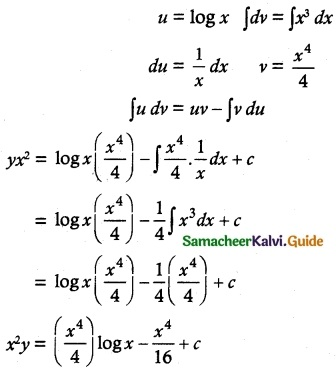Multiply by 16
16x²y = 4x4 log x – x4 + 16c is a required solutionQuestion 15.
$$\frac { dy }{ dx }$$ + $$\frac { 3y }{ x }$$ = $$\frac { 1 }{ x^2 }$$, given that y = 2 when x = 1
Solution:
The given differential equation can be written as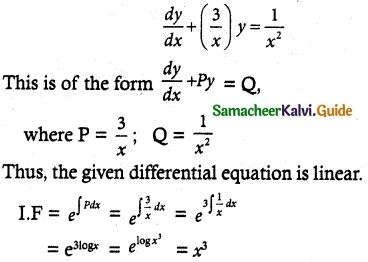So, its solution is given by
y × I.F = ∫(Q × I.F) dx + c
yx³ = ∫ $$\frac { 1 }{ x^2 }$$ + x³ dx + c
= ∫x dx + c
y x³ = $$\frac { x^2 }{ 2 }$$ + c
2yx³ = x² + c
Given that y = 2 when x = 1
2 (2) (1)³ = 1 + c
4 – 1 = c
c = 3
∴ 2yx³ = x² + 3 is a required solution.